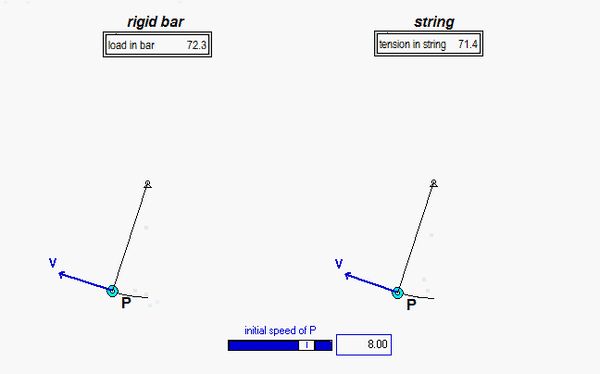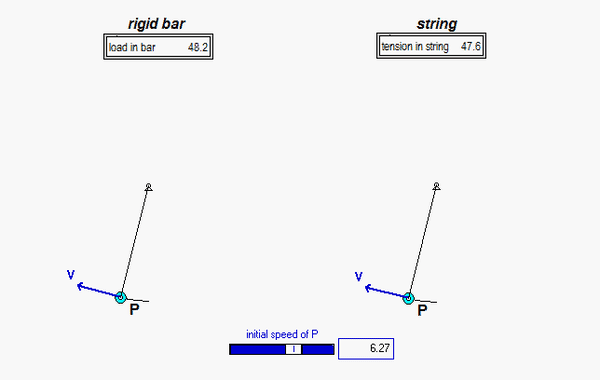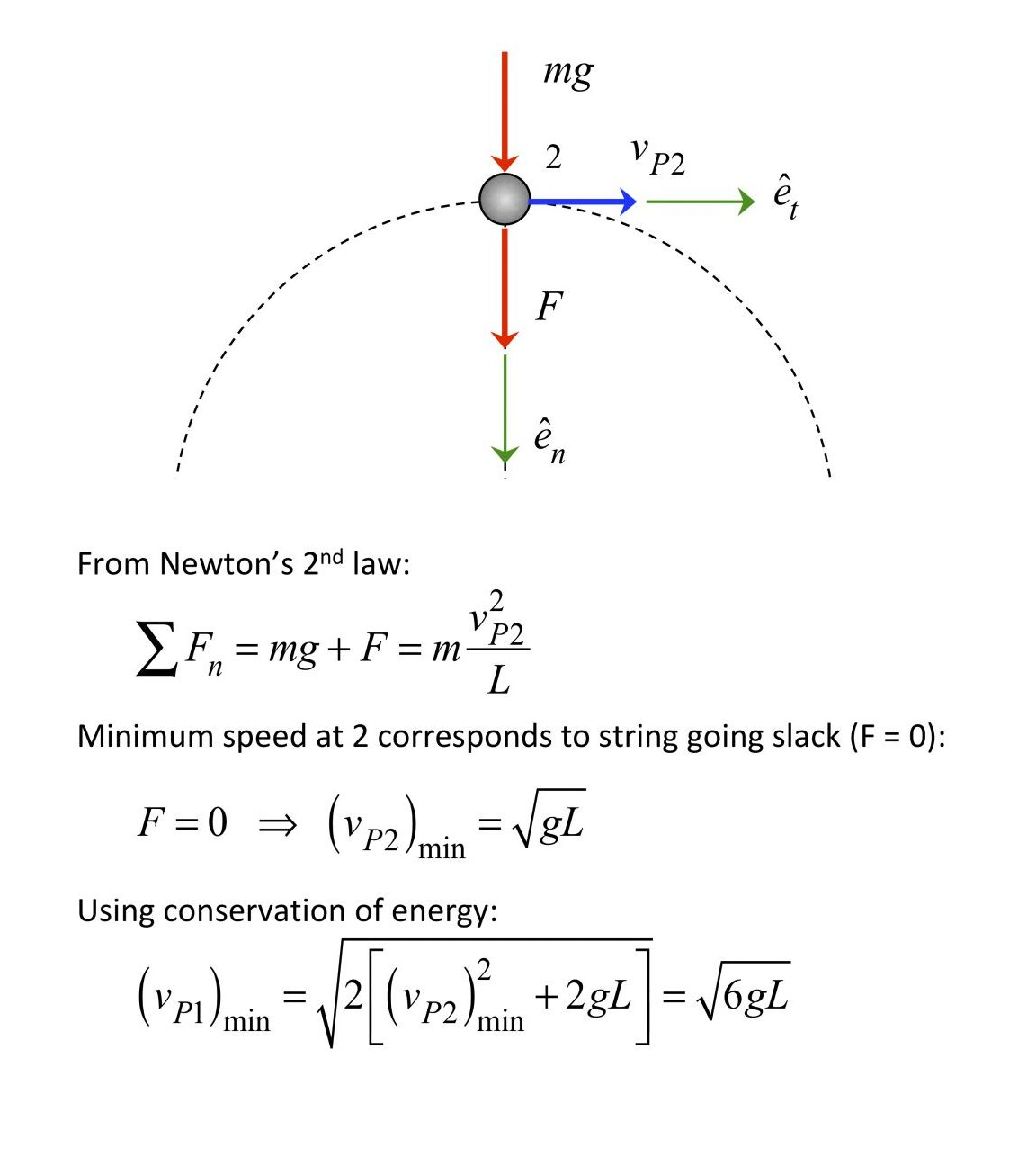# You can't push on a string

System I shown below is made up of a particle of mass P connected to a pin joint at O with a rigid bar having negligible mass. Particle P is given a velocity to the left with a speed of vP1 when at position 1. System II is identical to System I, except that the rigid bar is replaced by a string.For each system, it is desired to determine the minimum value of speed for P at position 1, (vP1)min in order for P to reach the top position at 2. For an initial speed larger than these minimum values, the motions of the two systems are as shown below. As expected, the paths of P in both systems is circular, with the load carried by the bar/string being in tension.System I
For System I, (vP1)min will correspond to vP2 = 0. Using conservation of energy, it can be shown that (vP1)min = √(4gL).
The motions for the two systems using this minimum value for the initial speed of P are shown below. As expected, System I with the rigid bar has P reaching the top position with nearly zero speed. Near this top position the bar is in compression. At this speed, System II with the string behaves differently: the string goes slack (since the string cannot sustain compression), and P never reaches the top position. Therefore a larger value for (vP1)min  is needed for the string.System II
Based on the above observation, the value of (vP1)min for System II will need to be larger than that for System I. How do we determine this minimum speed? See the following.Here we used Newton's 2nd law to determine the relationship required between the string tension and the speed of P. The minimum speed for P at the top occurs when the string goes slack, or F = 0. Then using the work/energy equation, we find the minimum speed of P at the bottom.